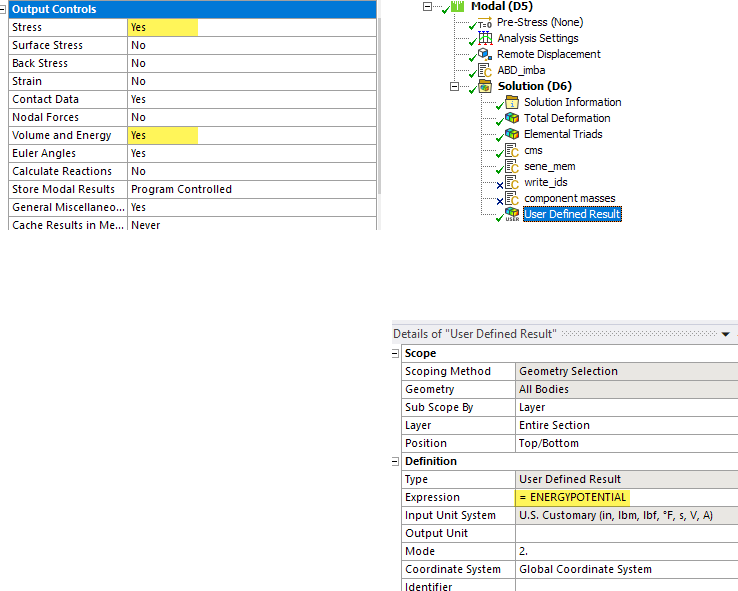## General Mechanical

•vibrachid
Subscriber

i want to calculate the starin modal energy using ansys workbench.

is the potential energy and the kinitic energy the strain modal energy ?.

I carried out the modal analysis, afterwards I carried out the harmonic analysis (solution) and under the harmonic analysis tab I calculated the potential energy + kinetic energy and the result is zero (potential energy + kinetic energy) = 0 on the whole body, why? where did I make the mistake ?.

•peteroznewman
Subscriber
nRead this article: https://medium.com/swlh/energize-your-ansys-dynamic-mechanical-analyses-b94c58da9a30nIt was written by  and posted in this Discussion: https://forum.ansys.com/discussion/comment/96298#Comment_96298nYou got zero because you did not turn on Volume and Energy in the Output Requests. nENERGYPOTENTIAL alone is the Strain Energy.n